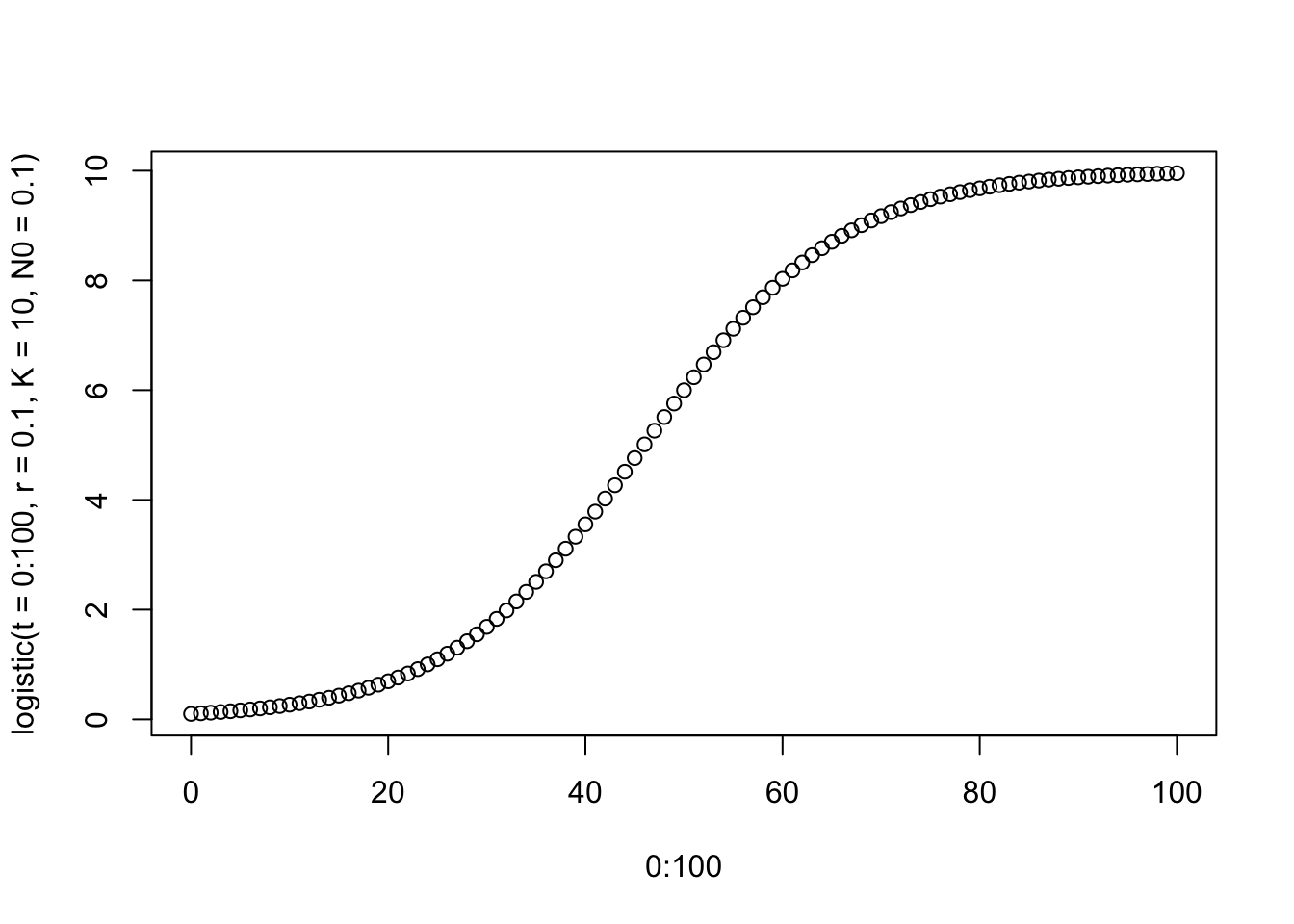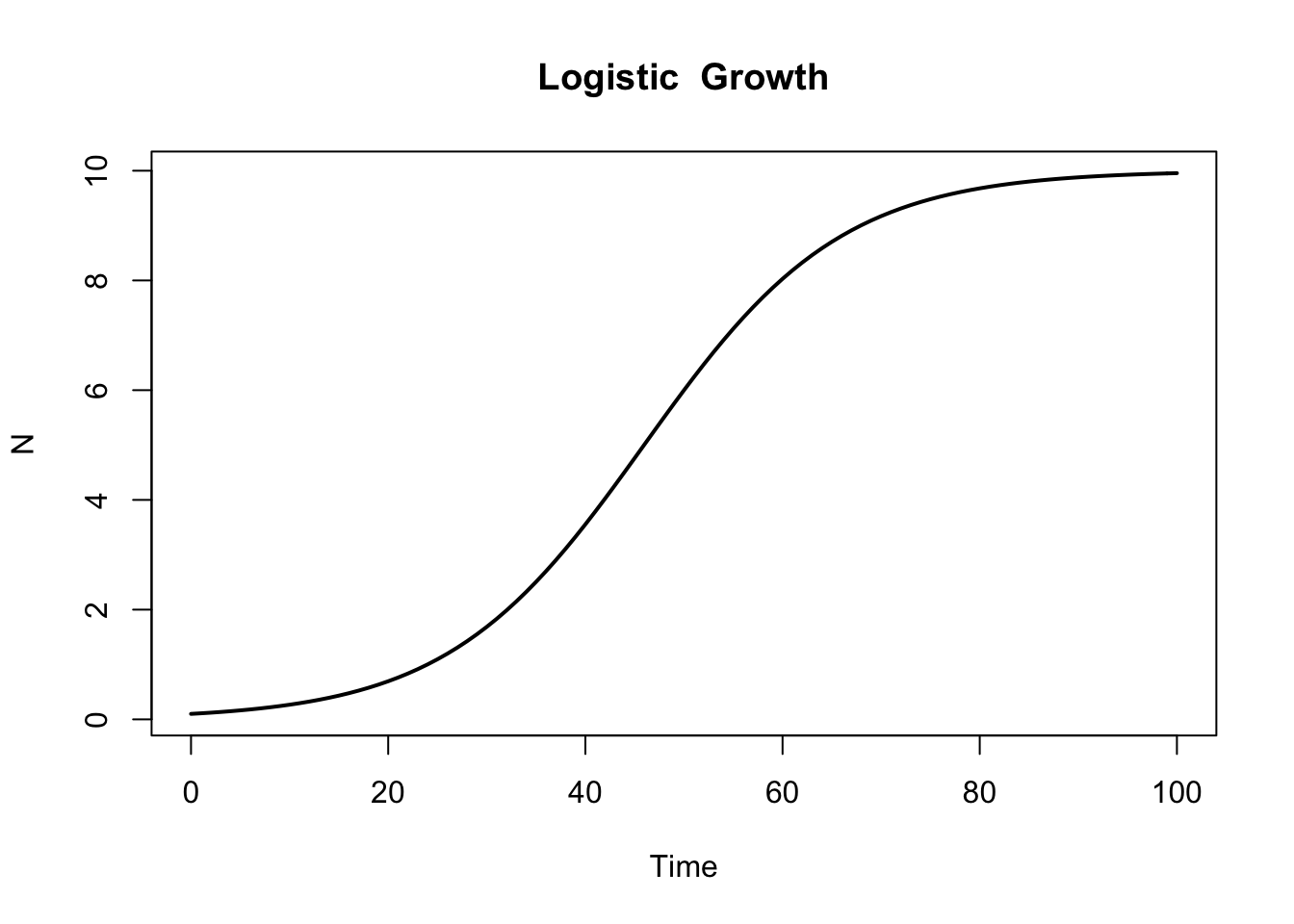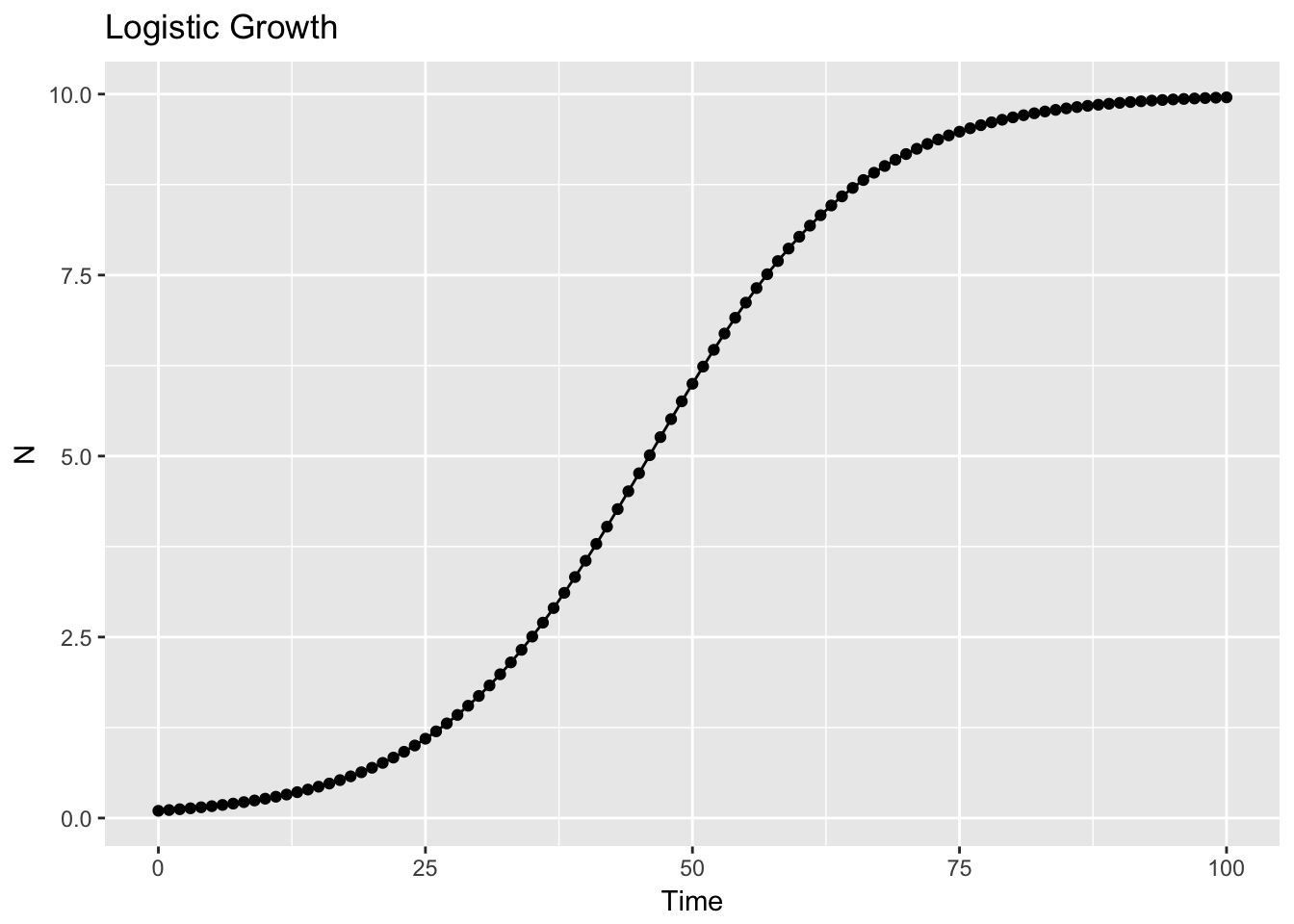## Overview

Our work has taken us into the realm of Ordinary Differential Equations. This tutorial is an exploration into how to plot output from these models - both for simulations (to increase understanding of how the models work), and for reporting of empirical results. Much is borrowed directly from http://desolve.r-forge.r-project.org/slides/tutorial.pdf.

## Outline

In this session we cover …

A. Analytic Solution of Differential Equation
B. Numerical Solution of Differential Equation (4 Steps)
C. Expansion to Multiple Scenarios (Persons)
D. Oscillator Model Example (VanDerPol Equation)
E. Oscillator Model Example (Univariate Linear Oscillator)
F. Bivariate System - Predator Prey Model
G. Bivariate System - Linear Oscillator Model

library(deSolve)  #for the differential equation solvers
library(ggplot2)   #for plotting

## A. Analytic Solution of Differential Equation

Let’s start with a very simple model - the logistic growth model. In differential form, the model is … (using the notation often used in ecology) …
$\frac{dN}{dt} = rN\left( \frac{1 - N}{K} \right)$

The analytical solution is derived by integrating and is … $N = \frac{KN_0exp(rt)} {(K + N_0(exp(rt) - 1))}$

(see Ram & Grimm, 2015 for discussion from a growth curve modeling perspective).

We can make a function of the integrated “solution” and plot a simulated curve.

logistic <- function(t, r, K, N0){
K * N0 * exp(r*t) / (K + N0 * (exp(r*t) - 1))
}
plot(0:100, logistic(t = 0:100, r = 0.1, K = 10, N0 = 0.1))## B. Numerical Solution of Differential Equation (4 Steps)

We can also use the deSolve library to obtain a numerical solution. Four steps are needed.

#### 1. Write the differential equation as a function.

difflogistic <- function(time, y, parms){
with(as.list(c(y,parms)),{
dN <- r * N * (1 - N / K)
list(dN)
})
}

#### 2. Specify vectors with the initial values, model parameters, and times over which to integrate.

#Initial Values
initvalues.1 <- c(N = 0.1)
#Model Parameters
parms.1 <- c(r = 0.1, K = 10)
#Times over which to integrate
times <- seq(0, 100, 1)

#### 3. Solve the differential equation using numerical methods.

This is done using the ode() function, which is itself a wrapper of the lsoda() function (and a collection of similar functions for different types of integrations (e.g., Runge-Katta), some of which work better with particular types of models (e.g., stiff and non-stiff systems)).

out <- ode(y = initvalues.1, times = times, func = difflogistic, parms = parms.1) 

This produces an object of class deSolve that can then be examined, summarized, used for diagnosis, and plotted.

head(out)
##      time         N
## [1,]    0 0.1000000
## [2,]    1 0.1104022
## [3,]    2 0.1218708
## [4,]    3 0.1345160
## [5,]    4 0.1484538
## [6,]    5 0.1638111
summary(out)
##                  N
## Min.      0.100000
## 1st Qu.   1.095729
## Median    5.998616
## Mean      5.395638
## 3rd Qu.   9.480876
## Max.      9.955256
## N       101.000000
## sd        3.902511
diagnostics(out)
##
## --------------------
## lsoda return code
## --------------------
##
##   return code (idid) =  2
##   Integration was successful.
##
## --------------------
## INTEGER values
## --------------------
##
##   1 The return code : 2
##   2 The number of steps taken for the problem so far: 105
##   3 The number of function evaluations for the problem so far: 211
##   5 The method order last used (successfully): 5
##   6 The order of the method to be attempted on the next step: 5
##   7 If return flag =-4,-5: the largest component in error vector 0
##   8 The length of the real work array actually required: 36
##   9 The length of the integer work array actually required: 21
##  14 The number of Jacobian evaluations and LU decompositions so far: 0
##  15 The method indicator for the last succesful step,
##            1=adams (nonstiff), 2= bdf (stiff): 1
##  16 The current method indicator to be attempted on the next step,
##            1=adams (nonstiff), 2= bdf (stiff): 1
##
## --------------------
## RSTATE values
## --------------------
##
##   1 The step size in t last used (successfully): 1
##   2 The step size to be attempted on the next step: 1
##   3 The current value of the independent variable which the solver has reached: 100.8645
##   4 Tolerance scale factor > 1.0 computed when requesting too much accuracy: 0
##   5 The value of t at the time of the last method switch, if any: 0
## 

These diagnostics output may be useful for checking the kind of integration method used (ode() chooses on its own), and if there were problems. (I have not run enough of these yet to know how, specifically, to use the diagnostics)

#### 4. Plot the output trajectory (solution).

plot(out, main="Logistic  Growth", lwd=2, xlab="Time", ylab="N")Given our proclivity for plotting using ggplot2 function, we can also make the plot there, after conversion of the deSolve object into a data frame.

outplot=as.data.frame(out)

ggplot(data=outplot, aes(x=time, y=N)) +
geom_point() +
geom_line() +
xlab("Time") +
ylab("N") +
ggtitle("Logistic Growth")## C. Expansion to Multiple Scenarios (Persons)

We can expand by plotting multiple scenarios (e.g., simualted trajectories for a set of selected or randomly chosen parameters (or starting values)).

#Use a list if many unknown number of outputs
outlist <- list()
plist <- cbind(r = runif(30,min=0.1,max=5),
K = runif(30,min=8,max=15))
#Loop through the matrix of parameter values
for(i in 1:nrow(plist)){
outlist[[i]] <- ode(y = initvalues.1, times = times, func = difflogistic, parms = plist[i,])
}
plot(out,outlist, lwd=2)